Vibrations Index Page

Free Vibrations (Single Degree of Freedom )

Symbols..... Simple Harmonic Motion..... Free Natural Vibrations..... Damped Vibrations..... Critical Damping.....
Underdamped oscillations..... Overdamped oscillations..... Logarithmic Decrement..... Angular Vibrations.....

Symbols

 x = distance in x direction (m) dx/dt = velocity in x direction (m/s) d 2x/dt2 = acceleration in x direction (m/s2) v = dx/dt = velocity (m/s) f = d 2x/dt2 = acceleration (m/s2) θ = angular rotation (radians) dθ /dt = angular velocity (radians /s) d 2θ /dt2 = angular acceleration (radians /s 2) α= d 2θ /dt2 = angular acceleration (radians /s 2) m = mass ( kg) I = Moment of Inertia ( kg .m2) k = spring stiffness (N/m) c = coefficient of viscous damping (N s /m) cc = critical damping coefficient.(N s /m) ζ = Damping ratio = c / (2 m ωn ) = c / [ 2.m √ (k/m)] π = pi ω = angular velocity (radians /s) ωn = Natural frequency (radians /s)

Simple Harmonic Motion

Generally free natural vibrations occur in elastic system when a body moves away from its rest position.   The internal forces tend to move the body back to its rest position.   The restoring forces are in proportion to the displacement.   The acceleration of the body which is directly related to the force on the body is therefore always towards the rest position and is proportional to the displacement of the body from its rest position.  The body moves with simple harmonic motion...

Simple harmonic motion is most conveniently shown as the projection on the vertical (x) axis of a point rotating in a circular motion (radius a) at a constant angular velocity ω.

The tangential velocity of the point = ω a.
The acceleration of the rotating point toward the centre of the circle..= ω 2. a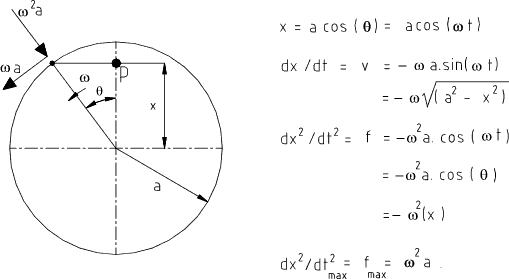The time taken to complete on cycle = t p = 2. π / ω The rotating speed (frequency of vibration) n = 1 / t p = ω / 2 . π The acceleration dx 2 / dt 2 = f = ω 2 . x and therefore ω = Sqrt ( f /x ) Therefore n = Sqrt ( f / x )   . 1 / ( 2 π ) ..

Free Natural Vibrations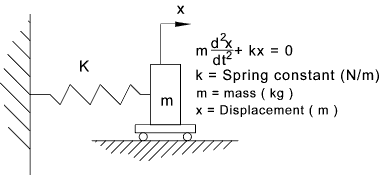The extension of the spring results in a restoring force of F = k.x The mass will accelerate at a rate directly proportional to the restoring force The equation of motion of the above spring/mass system is therefore...m .dx2 / dt 2 = - k . x This may be written as dx2 /dt 2 + ω n2 . x = 0 ....... ( after making ωn 2 = k / m ) The "Natural circular frequency" = ωn ....... (units = rads/s) Then "Natural frequency"   n   =   ω n ( 2 π )   =  Sqrt (k / m ) / ( 2 π ).....(units = cycles/s)

Damped Vibrations ..(Viscous Damping)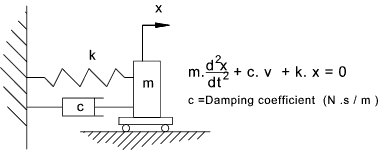The resisting force of the damper is directly proportional to the velocity of the mass

The equation of motion of the above damper/spring/mass system is therefore

m.dx2 /dt 2 = - k.x - c.dx /dt

This equates to the general expression of the equation of motion of a ocillating system with damping

m.dx2 /dt 2 + c.dx /dt + k.x = 0
dividing throughout by m
dx2 /dt 2 + (c/m).dx /dt + (k/m ) .x = 0

Now by making ωn 2 = k / m
and by making ζ = c / (2 m ωn ) = c / [ 2.m (k/m)]
the following version of the equation results

dx2 /dt 2 + 2ζ ωn dx /dt + ωn2 x = 0

Note: ζ is called the damping ratio and is described a little better under heading critical damping ratio below.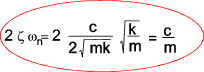a bit complicated but simplifies the final analysis

The equation is solved by assuming a solution of the form x = e s.t       (s = constant)

Substituting this relationship into the equation results in the formula

( s 2 + (c/m)s + (k/m) ) e st = 0 = ( s 2 + 2ζ ωns + ωn2) e st

Therefore ;

s 2 + s (c/m) + k/m = 0 = s 2 + 2ζ ωn s + ωn2

This equation has two roots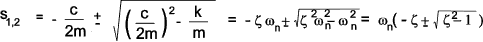The solution of the equation in general forms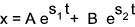A and B are constants which can be evaluated from initial values of x and dx/dt..
The substitution of the roots into the general form results in the formula .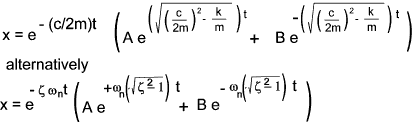The term e - ( c / 2 m) t represents an exponentially decaying factor...The expression inside the brackets can have three general results which significantly affect the solution.

 1).. if (c /2 m) 2 = (k / m) .. (ζ 2 = 1 )   the factor inside the bracket is 0 and the solution is critically damped 2).. if (c /2 m) 2 < (k / m).. (ζ 2 < 1 )  the factor inside the bracket is negative : the solution is underdamped 3).. if (c /2 m) 2 > (k / m).. (ζ 2 > 1 )  the factor inside the bracket is positive and the solution is overdamped 4)..if (c /2 m) 2 = 0 ..(ζ 2 = 0 )The system is undamped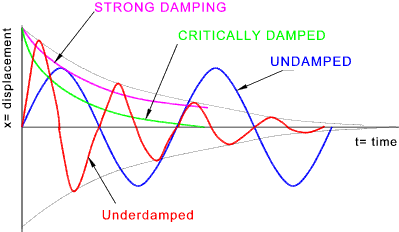Critical damping ...( (c /2 m) 2 = (k / m) and ζ= 1 (see below) )

The damping value c which results in [ (c/2m) 2 - (k/m) = 0 ] is called the critical damping coefficient cc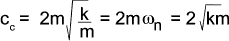The damping can be expressed in terms of the damping ratio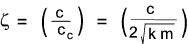This results in the values..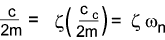Underdamped Oscillations.. ( ζ <1 or (c /2 m) 2 < (k / m))

In the underdamped case the square root of a negative number is an imaginary number..resulting in roots of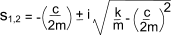This results in a oscillatory solution of the form..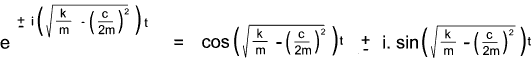The frequency of damped oscillation (rads/s) is equivalent to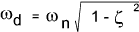The equation of motion for the underdamped case ( ζ < 1 ) is rewritten as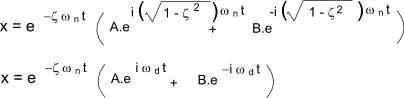This equation can be transformed to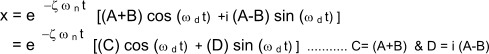This equation can be further transform into an equation with one trigonometric term as follows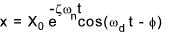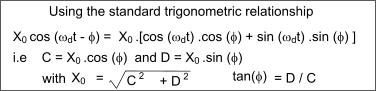Overdamped Oscillations ....( ζ > 1 and (c /2 m) 2 > (k / m))

The equation of motion for the overdamped case ( ζ > 1 )is rewritten as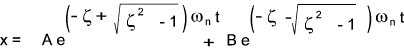The equation of motion for the critally damped case ( ζ = 1 )is written as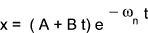Logarithmic Decrement....

The rate at which the amplitude of vibrations decays over time provides a very useful method of identifying the degree of damping. See the figure below for the plot of a typical underdamped vibration.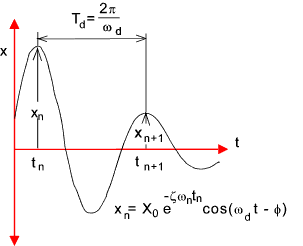The equation for of motion for underdamped oscillations arrived at above can be used to establish the amplitude of any of the cycles. i.e.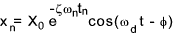Considering the amplitudes of successive cycles when cos(ω dt - φ) = 1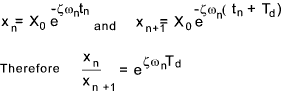The logarithmic decrement δ is ln( xn / xn+1 ).. Normally n = 1 and n+1 is therefore 2.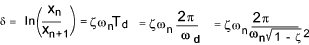The damping ratio ζ can be expressed in terms of the logarithmic decrement δ as follows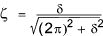It is clearly possible to determine the damping ratio experimentally for a mechanical system be initiating vibrations and measuring the amplitude of the vibrations.

Angular Vibrations

Consider a disc with a moment of Inertia I suspended on a vertical bar with a torsional stiffness q with a viscous damper attached with a viscous damping coefficient c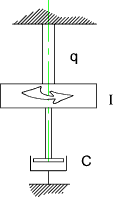The equation of motion of this system is expressed by the equation ..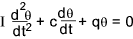This equation can be expressed using the natural circular frequencey ωn and the damping ratio ζ..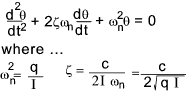It is clear that this equation is the same as the equation of motion for linear motion as reviewed above and can be analysed using the same principles

 Links on Free Vibrations Simple Harmonic Motion ...Animations illustrating the features of this phenomenon Hyperphysics ...Very clear Notes on simple harmonic Motion Theory Of Vibrations ...Excellent easy to follow notes Applet Damped Ocillator ...Very good for trying out damping and stiffness values Applet Damped Ocillator ...Very good for trying out damping and stiffness values ..Basic Theory of Vibrationss...Chapter two of a text book by RE Blake
Vibrations Index Page